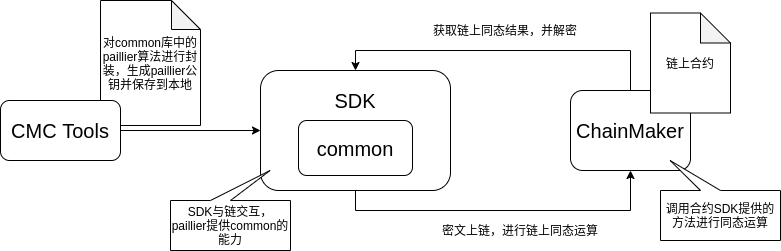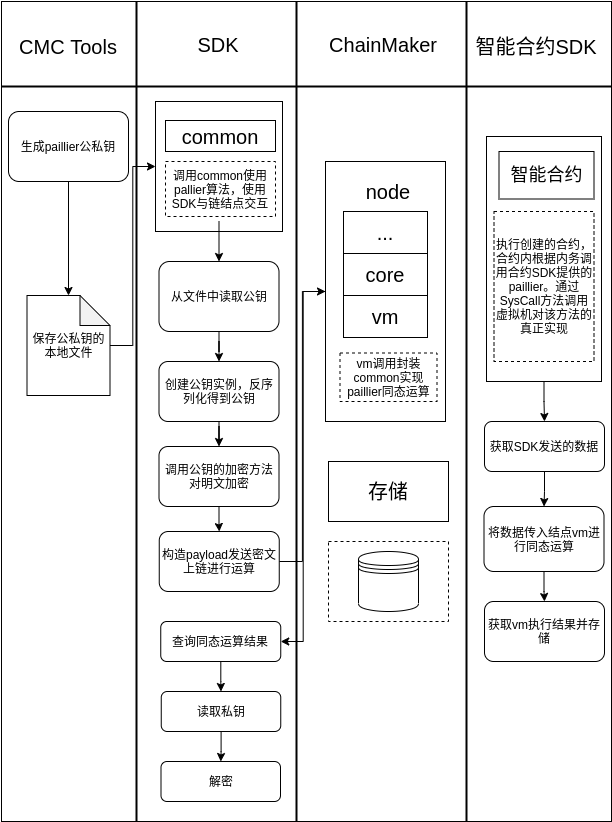# 长安链同态加密方案设计文档¶

## 背景¶

### 需求¶

#### 具体场景¶

• 结合区块链中的智能合约和同态加密技术，在个人征信隐私保护中，利用同态加密，设置访问权限，在不暴露个人明文信息的同时，创建与之相匹配的合约，但征信系统无法得知访问用户的需求。

• 强调隐私的业务，例如机构之间转账，隐藏账户余额，将用户账户余额进行加密后，通过密文之间的加减计算，进行隐秘的转账。

### Paillier加密算法¶

#### Paillier算法概述¶

Paillier算法是公钥加密体系的一个代表算法。但是，不同于传统的公钥加密算法，Paillier 算法除了实现对数据的公钥加密，还能保证在密文上直接进行加法操作，其结果解密后与在明文上进行操作的结果一样，因此Paillier算法完全的满足密文的加法同态性。除此之外，Paillier算法还支持明文和密文的乘法操作，具有良好的同态性，相比于当前的其他同态加密更加实用。

##### 算法流程¶

Paillier加密算法定义与一般公钥加密相同，主要由以下三个部分组成：

• $$\mathsf{KeyGen}(\lambda) \rightarrow (pk, sk)$$：密钥生成算法，由KGA运行，输入为安全参数$$\lambda$$，输出为一对公钥私钥对$$(pk,sk)$$

• $$\mathsf{Encrypt}(m,pk) \rightarrow CT$$：加密算法，由加密方运行，输入为用户公钥$$pk$$，明文空间的任一消息$$m$$，输出对应密文 $$CT$$

• $$\mathsf{Decrypt}(sk,CT) \rightarrow m$$：解密算法，由解密方运行，输入为解密私钥$$sk$$，密文$$CT$$，输出对应明文$$m$$

##### 同态性质¶

​ 同态加密是指这样一种加密函数，对明文进行环上的加法和乘法运算再加密，与加密后对密文进行相应的运算，结果是等价的。由于这个良好的性质，人们可以委托第三方对数据进行处理而不泄露信息。具有同态性质的加密函数是指两个明文$$a、b$$满足$$Dec(En(a)⊙En(b))=a⊕b$$的加密函数。其中$$En$$是加密运算，$$Dec$$是解密运算，$$⊙、⊕$$分别对应明文和密文域上的运算。当$$⊕$$代表加法时，称该加密为加同态加密：当$$⊕$$代表乘法时，称该加密为乘同态加密。• 加法同态性

$$\mathsf{Encrypt}(m_1)*\mathsf{Encrypt}(m_2) = \mathsf{Encrypt}(m_1+m_2)$$ ;

• 明文和密文乘法同态性

$$\mathsf{Encrypt}(m_1)^{m_2} = \mathsf{Encrypt}(m_1*m_2)$$ ;

#### Pailier算法研究现状¶

##### 研究进展¶

Paillier1在1999年提出了一种新的同态加密算法，即Paillier加密算法，由于Paillier算法可以进行有效的加法同态，受到了广泛的关注。2001 年，Damgard 和 Jurik 2 使用模$$n^i$$进一步对 Paillier加密体制进行了推广，并对其应用进一步推广。 Choi等人3通过选取特殊的参数$$g$$$$(g^λ =1+ n\mod n^2，λ = lcm( p −1,q −1))$$改进了 Paillier加密体制。 但是Choi 等提出的变体方案不能抵抗选择密文攻击。在2006 年的欧密会上，Schoenmakers和Tuyls 4$$x∈Z_n$$的Paillier 加密方案，扩展到了对$$x$$进行逐比特加密的Pailier加密方案。2013 年的欧密会上，Joye 和Libert 5 利用$$2^k$$阶剩余问题进一步对Paillier 加密方案进行了推广。在2014年的亚密会上，Catalano 6等等人则是提出了支持Paillier加密实例的外包密文的公开可验证授权计算方案。Castagnos Laguilaumie 7在2015年设计了一种线性同态加密方案，其安全性依赖于判定Diffie-Hellman困难问题。

​ 表1 经典同态算法对比

Paillier算法

RSA 算法

Gentry 算法

### 采用的paillier同态加密算法¶

#### 基本概念¶

$$Paillier$$加密系统是一种加法同态公钥加密系统，这种加密技术已广泛应用于加密信号处理或第三方数据处理领域。其同态特性表现为:在加密后可直接对密文进行相应的算术运算，其运算结果与明文域中对应的运算结果一致。其概率特性表现为:对于相同的明文，可通过不同的加密过程得到不同的密文，从而保证了密文的语义安全。

#### 前提假设​：¶

1. 随机选择两个大质数p、q满足$$gcd(pq, (p-1)*(q-1))$$​。

2. $$计算n=p*q$$

3. $$g = n+1$$

4. $$λ = φ(n) = (p-1)*(q-1)$$

5. 定义$$L(x) = (x-1) / n$$

6. $$μ = φ(n)^{-1} (mod{n})$$

7. 公钥为$$(n，g)$$

8. 私钥为$$(λ，μ)$$

#### Encryption¶

1. $$m$$为要加密的消息，显然需要满足，$$0 \leq m < n$$

2. 选择随机 $$r$$，保证$$gcd(r, n) = 1$$

3. 密文$$c$$$$c = (g^m) *(r^n) mod n^2$$

#### Decryption¶

1. $$m = ( L( c^λ mod n^2 ) * μ ) mod n$$

$$L(c^λ (mod{n^2}) * μ) (mod{n})$$

$$L(g^{mλ}*r^{nλ} pmod{n^2}*μ) (mod{n})$$

$$r^{nλ} = r^{n(p-1)*(q-1)} = r^{φ(n^2)}$$

①式 => $$L(g^{mλ} pmod{n^2}*μ) pmod{n}$$

$$g = n+1$$

$$g^{mλ} = (1+n)^{mλ} \equiv nλ+1 (mod{n^2})$$

②式=> $$L((nmλ+1)*μ) mod{n}$$

=> $$\frac{(nmλ+1)-1}{n}*μ (mod{n})$$

=>$$(mλ*μ) (mod{n})$$

$$λ = φ(n)，μ = φ(n)^{-1} (mod{n})$$

∴③式： $$(mλ*μ) \equiv m (mod{n})$$

#### 同态加法¶

$$D\left(E\left(m_{1}, r_{1}\right) \cdot E\left(m_{2}, r_{2}\right) \bmod n^{2}\right)=m_{1}+m_{2} \bmod n$$

$$\begin{array}{l}D\left(E\left(m_{1}, r_{1}\right) \cdot E\left(m_{2}, r_{2}\right) \bmod n^{2}\right)=D\left(g^{m_{1}} \cdot r_{1}^{n} \cdot g^{m_{2}} \cdot r_{2}^{n}\right) \bmod n^{2}=D\left(g^{m_{1}+m_{2}} \cdot\left(r_{1} r_{2}\right)^{n}\right) \bmod n^{2}=m_{1}+m_{2}\end{array}$$

#### 同态数乘¶

$$D\left(E\left(m_{1}, r_{1}\right)^{k} \bmod n^{2}\right)=k m_{1} \bmod n$$

$$D\left(E\left(m_{1}, r_{1}\right)^{k} \bmod n^{2}\right)=D\left(\left(g^{m_{1}} \cdot r_{1}^{n}\right)^{k}\right) \bmod n^{2}=D\left(g^{k m_{1}} \cdot r_{1}^{k} n\right) \bmod n^{2}=k m_{1} \bmod n$$

## 方案描述¶

### 整体架构¶### 执行流程¶## 参考¶

 Paillier P. Public-Key Cryptosystems Based on Composite Degree Residuosity Classes[J]. Lecture Notes in Computer Science, 1999, 547(1):223-238.

 Damgård I, Jurik M. A Generalisation, a Simplication and Some Applications of Paillier’s Probabilistic Public-Key System[J]. Lecture Notes in Computer Science, 2001, 7(45):119-136.

 Choi D H, Choi S, Won D. Improvement of Probabilistic Public Key Cryptosystems Using Discrete Logarithm[C]. International Conference Seoul on Information Security and Cryptology. Springer-Verlag, 2001:72-80.

 Schoenmakers B, Tuyls P. Efficient Binary Conversion for Paillier Encrypted Values[C]. Advances in Cryptology - EUROCRYPT 2006:522-537.

 Joye M, Libert B. Efficient Cryptosystems from 2k-th Power Residue Symbols[M]. Advances in Cryptology – EUROCRYPT 2013. Springer Berlin Heidelberg, 2013:651-4.

 Catalano D, Marcedone A, Puglisi O. Authenticating Computation on Groups: New Homomorphic Primitives and Applications [M]. Advances in Cryptology – ASIACRYPT 2014. 193-212.

 Castagnos G, Laguillaumie F. Linearly Homomorphic Encryption from DDH [M]// Topics in Cryptology –- CT-RSA 2015. Springer International Publishing, 2015:487-505.-